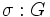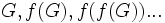# Subgroup-defining function

BEWARE! This term is nonstandard and is being used locally within the wiki. [SHOW MORE]

## Definition

### Symbol-free definition

A subgroup-defining function is a rule that sends each group to a subgroup, and such that any isomorphism of groups take the defined subgroup of one group to the defined subgroup for the other.

### Definition with symbols

A subgroup-defining function is a rule$f$ that sends each group$G$ to a subgroup$f(G)$. By subgroup here we mean an abstract group along with an embedding into$G$. The subgroup-defining function should satisfy the property that whenever there is an isomorphism$\sigma: G$$H$, the image of$f(G)$ under$\sigma$ is$f(H)$.

## For particular kinds of groups

Subgroup-defining functions of particular kinds on groups of prime power order are particularly important. A subgroup-defining function on all groups whose order is a power of$p$, for a fixed prime$p$, that returns nontrivial subgroups for nontrivial groups, is termed a characteristic p-functor. Characteristic p-functors are a special case of conjugacy functors.

## Property theory

### Subgroup properties satisfied

Further information: subgroup-defining function value is characteristic

Any subgroup-defining function defines a characteristic subgroup. If the function is a verbal function, its defines a verbal subgroup and hence a fully characteristic subgroup, whereas if the function is a word-bound function, it defines a word-bound subgroup and hence a strictly characteristic subgroup.

If the subgroup-defining function is monotone and idempotent, then it is intermediacy-preserved. Thus, if it always satisfies a subgroup property$p$, it also always satisfies the property intermediately$p$. In particular, any subgroup obtained this way is an intermediately characteristic subgroup.

## Associated constructions

### Quotient-defining function

To any subgroup-defining function, we can also associate a corresponding quotient-defining function, which sends a group to the quotient group by that subgroup. Note that the quotient group is well-defined because a subgroup-defining function always returns a characteristic subgroup, and every characteristic subgroup is normal.

### Descending series

Given a subgroup-defining function, the associated descending series, also called the series obtained by iteration of the subgroup-defining function, is the series that, for any group$G$, gives$G, f(G), f(f(G))...$.

We can continue this series transfinitely by defining the$(\alpha +1)^{th}$ term as$f$ of the$\alpha^{th}$ term, and by defining the term for a limit ordinal as the intersection of all its predecessors.

As with any descending series-defining function, we can talk of the length, and the notion of being descendable to the trivial subgroup. Thus, we can associate these notions to any subgroup-defining function.

### Ascending series

Given a subgroup-defining function, the associated ascending series, also called the series obtained by quotient-iteration, is defined as PLACEHOLDER FOR INFORMATION TO BE FILLED IN: [SHOW MORE]

As with any ascending series-defining function, we can talk of the length, and the notion of being ascendable to the whole group. Thus, we can associate these notions to any subgroup-defining function.

## Operators to group properties

### The image operator

The image operator is a map from the collection of subgroup-defining functions to the collection of group properties that sends a subgroup-defining function to the property of being a group that can arise as the result of applying the subgroup-defining function to some group.

### The fixed-point operator

The fixed-point operator is a map from the collection of subgroup-defining functions to the collection of group properties that sends a subgroup-defining function to the property of being a group which is fixed point under this subgroup-defining function.

For idempotent subgroup-defining functions, the fixed-point operator and image operator have the same effect.

### The free operator

The free operator is a map from the collection of subgroup-defining functions to the collection of group properties. This sends a subgroup-defining function to the property of being a group for which the corresponding subgroup is the trivial subgroup.